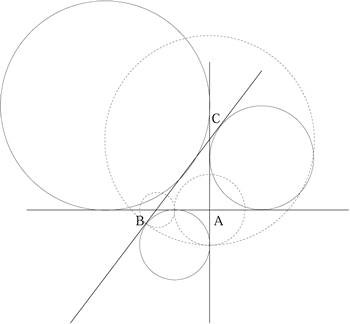#### You may also like### Baby Circle

A small circle fits between two touching circles so that all three circles touch each other and have a common tangent? What is the exact radius of the smallest circle?### Kissing

Two perpendicular lines are tangential to two identical circles that touch. What is the largest circle that can be placed in between the two lines and the two circles and how would you construct it?### Logosquares

Ten squares form regular rings either with adjacent or opposite vertices touching. Calculate the inner and outer radii of the rings that surround the squares.

# Escriptions

##### Age 16 to 18 Challenge Level:

This question uses the same method as the problem Polycircles from February 2000 and you might find it helpful to experiment with the interactive diagram.

The best solution to this problem came from Andrei Lazanu, age 13 of School No. 205, Bucharest, Romania. Can you finish off the solution?

I drew a right angled triangle, and circles having as centres the vertices of the triangle, and being tangent two by two at points on the sides of the triangle.

Let $r_A,\ r_B$ and $r_C$ be the radii of the touching circles centred at the vertices of the right angled triangle ABC, of sides $a,\ b$ and $c$, with the centres at the points A, B and C respectively. These radii satisfy the conditions:

\eqalign{ r_A + r_B &= c \cr r_B + r_C &= a \cr r_C + r_A &= b.}

This system of three equations with three unknowns could be solved in the following manner: adding all three equations, dividing the result by two and subtracting one by one the equations. I obtained:

\eqalign{ r_A &= {1\over 2}(b+c-a) \cr r_B &= {1\over 2}(a + c - b) \cr r_C &= {1\over 2}(a + b - c) \cr.}

I also drew the three escribed circles, as well as their points of intersection. I see that on each side, the points of intersection of the circles with centres at the vertices are the same as the points of tangency of the escribed circles with the sides. This can be explained by the fact that the lengths of the tangents to a circle, drawn from a point exterior to the circle, have equal lengths. This will enable us to find the radius of an escribed circle.Given any right angled triangle $ABC$ with sides $a,\ b$ and $c$, we have to find the radii of the three circles shown in the diagram with centres at $A,\ B$ and $C$ such that each circle touches the other two and two of the circles touch on $AB$ between $A$ and $B$, two circles touch on $CA$ produced and two circles touch on $CB$ produced.

The method is exactly as above but here we have

\eqalign{r_A + r_B &= c \cr r_C - r_A &= b\cr r_C - r_B &= a.}

By solving these equations and identifying which circles have equal radii you should be able to find the radius of one of the escribed circles.

The radii of the other escribed circles are found similarly.